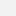Direkt zum InhaltDirekt zur SucheDirekt zur Navigation

# Humboldt-Universität zu Berlin - Mathematisch-Naturwissenschaftliche Fakultät - Institut für Mathematik

add legends to plots in r software the easiest way

## add legends to plots in r software the easiest way

Add legends to plots in R software : the easiest way! - Easy Guides - Wiki - STHDA

# R legend function

To add legends to plots in R, the R legend() function can be used. A simplified format of the function is :

``legend(x, y=NULL, legend, fill, col, bg)``

• x and y : the x and y co-ordinates to be used to position the legend
• legend : the text of the legend
• fill : colors to use for filling the boxes beside the legend text
• col : colors of lines and points beside the legend text
• bg : the background color for the legend box.

Example :

``````# Generate some data
x``````To avoid repeating the above R code, we can create a custom plot function as follow :

``makePlot``

# Title, text font and background color of the legend box

The arguments below can be used :

• title: The title of the legend
• text.font: an integer specifying the font style of the legend text; possible values are :
• 1: normal
• 2: bold
• 3: italic
• 4: bold and italic
• bg: background color of the legend box
``````makePlot()
# Add a legend to the plot
legend(1, 95, legend=c("Line 1", "Line 2"),
col=c("red", "blue"), lty=1:2, cex=0.8,
title="Line types", text.font=4, bg='lightblue')``````# Border of the legend box

The arguments box.lty, box.lwd and box.col can be used to modify the line type, width and color for the legend box border, respectively.

``````# Remove legend border using box.lty = 0
makePlot()
legend(1, 95, legend=c("Line 1", "Line 2"),
col=c("red", "blue"), lty=1:2, cex=0.8,
box.lty=0)
# Change the border
makePlot()
legend(1, 95, legend=c("Line 1", "Line 2"),
col=c("red", "blue"), lty=1:2, cex=0.8,
box.lty=2, box.lwd=2, box.col="green")``````# Specify legend position by keywords

The position of the legend can be specified also using the following keywords : `"bottomright", "bottom", "bottomleft", "left", "topleft", "top", "topright", "right" and "center".`

The effect of using each of these keywords are shown in the figure below :## Example 1: line plot

``````#  Example 1: line plot
makePlot()
legend("topleft", legend=c("Line 1", "Line 2"),
col=c("red", "blue"), lty=1:2, cex=0.8)``````## Example 2: box plot

``````attach(mtcars)
boxplot(mpg~cyl,
xlab="Cylinders", ylab="Miles/(US) gallon",
col=topo.colors(3))

legend("bottomleft", inset=.02, title="Number of Cylinders",
c("4","6","8"), fill=topo.colors(3), horiz=TRUE, cex=0.8)``````Note that the argument `fill` indicates the colors to use for filling the boxes beside the legend text

# Infos

This analysis has been performed using R statistical software (ver. 3.1.0).